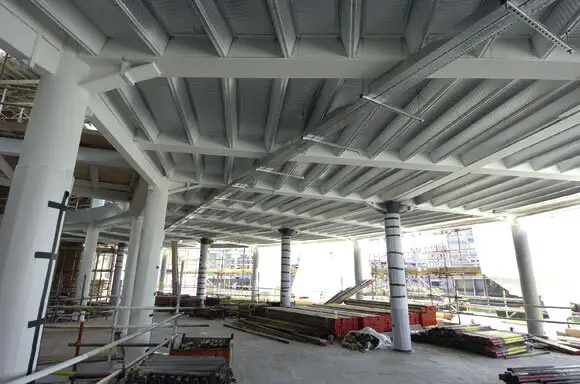# Design of Circular Hollow Section (CHS) Steel columnsCircular Hollow Sections (CHS) are round tubular steel sections that are used for a variety of purposes in civil engineering. They are usually available in the market as hot-rolled or cold-formed sections. Hot-rolled circular hollow sections are usually employed for structural purposes such as columns, struts, ties, etc.

Cold-formed sections are usually used as purlins and as framing for lightweight building construction. However, EN 1993-1-1 permits the use of both cold-formed and hot-rolled sections for structural purposes, even though the weldability of the former is usually a concern to structural engineers.

In offshore structures, CHS are preferred due to their capacity to minimise wave forces and for their structural efficiency. The structural efficiency of CHS members in bending, axial, and torsion is due to the uniform distribution of section materials about the polar axis. CHS is also known to offer a better strength-to-weight ratio in buildings and can lead to economical and lightweight construction.

The hollow in the member also offers the advantage of making provision for building services such as fire protection, ventilation, heating, etc. In terms of corrosion, CHS sections perform better than other open sections due to their round edges and smaller surface area. The aesthetic appeal of CHS members also makes them a preferred alternative to architects.

In this article, let us verify the axial load-carrying capacity of CHS members according to the provisions of EC3.

Solved Example

Assess the suitability of a hot-rolled 244.5 x 10 CHS in grade S355 steel as an internal column in a multi-storey building to resist an ultimate axial force of 2000 kN. The column has pinned boundary conditions at each end, and the inter-storey height is 4.5 m.

d = 244.5 mm
t = 10.0 mm
A = 7370 mm2
Wel,y = 415000 mm3
Wpl,y = 550000 mm3
I = 50730000 mm4

For a nominal material thickness (t = 10.0 mm) of less than or equal to 16mm the nominal value of yield strength fy for grade S355 steel is found from EN 10210-1 to be 355 N/mm2.

E = 210000 N/mm2

Section classification
ε = √(235/fy ) = √(235/355) = 0.81

Tubular sections (Table 5.2, sheet 3 of EN 1993-1-1):
d/t = 244.5/10 = 24.45

Limit for Class 1 section = 50ε2 = 32.8

24.45 < 32.8. Therefore section is Class 1

Resistance of the member to uniform compression (clause 6.2.4, EN 1993-1-1)
NC,Rd = (A.fy)/γmo = (7370 × 355) / 1.0 = 2616350 N = 2616 kN

NEd/NC,Rd = 2000/2616 = 0.764 < 1

Therefore section is okay for uniform compression.

Buckling resistance of member to compression (clause 6.3.1, EN 1993-1-1)

Nb,Rd = (χA.fy)/γmo for class 1, 2, and 3 sections

χ = 1/[Φ + √(Φ2 – λ2)] ≤ 1.0

Φ = 0.5 [1 + α(λ – 0.2) + λ2]

λ = √(Afy/Ncr)

Where;

Ncr = π2EI/Lcr2

Since the member is pinned at both ends, critical buckling length is the same for all axis Lcr = 4500mm

Ncr = (π2 x 210000 x 50730000)/45002 = 5192289.213 N = 5192 kN

λ = √(Afy/Ncr) = √(7370 x 355)/(5192 x 103) = 0.71

Selection of buckling curve and imperfection factor α
For a hot-rolled CHS, use buckling curve a (Table 6.2 of EN 1993-1-1).
For buckling curve a, α = 0.21 (Table 6.1 of EN 1993-1-1).

Buckling curves
Φ = 0.5 [1 + α(λ – 0.2) + λ2]
Φ = 0.5 [1 + 0.21(0.71 – 0.2) + 0.712 ] = 0.8056

χ = 1/[Φ + √(Φ2 – λ2)]
χ = 1/[0.8056 + √(0.80562 – 0.712)] = 0.842 < 1 ok

Therefore Nb,Rd = (χA.Fy)/γm1 = (0.842 × 7370 × 355) / (1.0 ) = 2202966.7 N = 2203 kN

NEd/Nb,Rd = 2000/2203 = 0.907 < 1

Therefore section is ok for buckling.

Summarily, the section is okay to resist axial load on it.